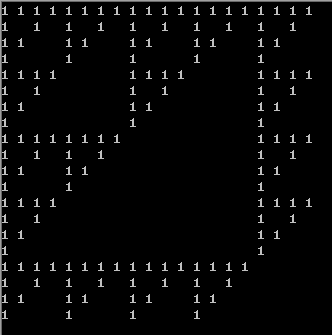• C语言-打印以下形式的数据：（找规律用数组输出，不能直接用下面给的数值初始话数组） ...1 4 9 16 25 4 9 16 25 1 9 16 25 1 4 16 25 1 4 9 25 1 4 9 16 方法一 观察数组，第一行，即1x1 2x2 3x3 4x4 5x5 k=(i+j)%5
C语言-打印以下形式的数据：（找规律用数组输出，不能直接用下面给的数值初始话数组）

打印以下形式的数据：（找规律用数组输出，不能直接用下面给的数值初始话数组）
1     4     9    16    25
4     9    16    25     1
9    16    25     1     4
16    25     1     4     9
25     1     4     9    16
方法一
观察数组，第一行，即1x1 2x2 3x3 4x4 5x5
k=(i+j)%5;a[i][j]=(k+1)*(k+1);
举例  k=0%5=0  a[i][j]=1
k=1%5=1 a[]=4
k=2%5=2 a[]=9
k=3%5=3 a[]=16
k=4%5=4 a[]=25

#include <stdio.h>
//k=(i+j)%5;a[i][j]=(k+1)*(k+1);
int main(void)
{
int a,i,j,k;
for(i=0;i<5;i++)
{
for(j=0;j<5;j++)
{
k=(i+j)%5;
a[i][j]=(k+1)*(k+1);
}
}

for(i=0;i<5;i++)
{
for(j=0;j<5;j++)
{
printf("%3d",a[i][j]);
}
printf("\n");
}
return 0;
}


方法二：一维数组

#include <stdio.h>
void main()
{
int a={1,4,9,16,25},i,j;

for(i=0;i<5;i++)
{//i表示第i行的输出
for(j=0;j<5;j++)
{
printf("%3d",a[(i+j)%5]);//下标运算，看作 {1,4,9,16,25}依次往左移
}
printf("\n");
}
}


方法三

#include<stdio.h>
int main(void)
{
int a,i,j;
for(i=0;i<5;i++)
{
for(j=0;j<5;j++)
{
if(i+j==4) a[i][j]=25;
if(i+j==3||i+j==8) a[i][j]=16;
if(i+j==2||i+j==7) a[i][j]=9;
if(i+j==1||i+j==6) a[i][j]=4;
if(i+j==0||i+j==5) a[i][j]=1;
}
}
for(i=0;i<5;i++)
{
for(j=0;j<5;j++)
{
printf("%4d",a[i][j]);
}
printf("\n");
}
return 0;
}



展开全文c语言
• 1、让我们开始在最上面的一行的中间放上1(在这个例中n=3) 你的任务是写一个程序去出哪个数会被放到右下角在n幻方中，当然，你可以使用上面的规律去构造幻方。 2、我们假定最后一行是第一行的上一行，向右上角移动...编程语言
• 题目： 有1、2、3、4个数字，能组成多少个互不相同且无重复数字的三位数？都是多少？ 【程序2】 题目：企业发放的奖金根据利润提成。利润(I)低于或等于10万元时，奖金可提10%；利润高 　于10万元，低于20万元时，...c++经典例题
• 规律是n^3-1(当n为偶数0，2，4) n^3+1(当n为奇数1，3，5) 答案：63 2.用两个栈实现一个队列的功能？要求给出算法和思路！ 设2个栈为A,B, 一开始均为空. 入队: 将新元素push入栈A; 出队: (1)判断栈B是否为空； (2)...C面试题
• 题意 对于一个（n+1）*（n+1）的矩阵，第一列和第一排为1，其他位置为1当且仅当上方和左方有一个1...1,3,7,9,17,21,25,27,43,51,59,63,71,75,81; 这种东西一般就求差再看看，就会发现：2,4,2,8,4,4,2,16,8,8,4,8,4...
题意
对于一个（n+1）*（n+1）的矩阵，第一列和第一排为1，其他位置为1当且仅当上方和左方有一个1，其他为0；
对于100%的数据，n<=1e9
题解
稍微画了一下，感觉从图像看不大出来，就去打了一个表：
1,3,7,9,17,21,25,27,43,51,59,63,71,75,81;
这种东西一般就求差再看看，就会发现：2,4,2,8,4,4,2,16,8,8,4,8,4,4,2
这样还看不大出来，再分析数据对于30%的数据n=2k-1，发现这个范围的答案就是3k,于是直觉就分出了组
2
4,2
8,4,4,2
16,8,8,4,8,4,4,2
那么就比较明显了吧，下面一排前一半是上一排*2，后一半就是上一排。
那么就想到一种做法，先把n分成2k+n'，就得到3k，剩下n'部分再递归处理，他是一个组的一部分，再这个组的前一半的部分就是上一个组的一半，在这个组后一半的部分直接递归。
注意记忆化就ok，可以用map记忆化，其实差不多，map还有查找速度，虽然数组只能只能开1e6。#include<bits/stdc++.h>
using namespace std;

#define ll long long
const int maxn=1000005;
ll n;
ll ans,f[maxn];

int get(int x){
int ret=-1;
while(x){
x>>=1;
ret++;
}
return ret;
}

ll qpow(ll a,ll b){
ll ret=1;
while(b){
if(b&1) ret*=a;
a*=a;
b>>=1;
}
return ret;
}

ll dfs(int s,int k){//第s组，前k项
if(s==1) return  2;
if(k<maxn&&f[s][k]) return f[s][k];
ll mid=(1<<(s-2)),ret;
if(k<=mid) ret=dfs(s-1,k)<<1;
else ret=dfs(s-1,k-mid)+(dfs(s-1,mid)<<1);
if(k<maxn) f[s][k]=ret;
return ret;
}

int main(){
freopen("c.in","r",stdin);
freopen("c.out","w",stdout);
scanf("%lld",&n);
n++;
int k=get(n);
ans+=qpow(3,k);
n-=(1<<k);
if(!n) {printf("%lld",ans);return 0;}
ans+=dfs(k+1,n);
printf("%lld",ans);
}

View Code
不过他们的规律好像和我不大一样，他们直接从答案入手，给出代码，具体原因之后再看。（code from ltw）#include<cstdio>
#include<algorithm>
#include<cstring>
#include<iostream>
#include<cmath>
#include<map>
using namespace std;
long long n;
map < int,long long > mp;
long long solve (long long x){
if (x==1) return 1;
if (x==2) return 3;
if (mp[x]) return mp[x];
return mp[x]=2*solve (ceil ((double)(x/2.0)))+solve (floor ((double(x/2.0))));
}
int main(){
freopen ("c.in","r",stdin); freopen ("c.out","w",stdout);
scanf ("%lld",&n);
printf ("%lld",solve (n+1));
}

View Code
还有看图像找规律的，三个1组成一个小三角形，再三个又是一个更大的。
先取一个lowbit（就是分解成2k+n'），然后再怎么搞搞就OK了。（咱也不知道，咱也不敢问）不过正解好像是数位DP？讲这个图形旋转45°就是杨辉三角？然后在利用Lucas定理？
话说今天数论都是打表搞的，这真是一个好方法。（掩饰自己不会数论）
顺便%%sxk  dalao，AK
转载于:https://www.cnblogs.com/sto324/p/11266357.html
展开全文• 一边长为a的直角三角形，a^2=c^2-b^2。可以发现1、491625...我们还可以发现，1、491625、36各项差为8、12、16、20，偶数的平方是4的倍数，那么c=a^2/4-1,b=a^2/4+1。 #include <iostream> us...

一边长为a的直角三角形，a^2=c^2-b^2。可以发现1、4、9、16、25依次差3、5、7、9...，所以任何一条长度为奇数的边a，a^2还是奇数，那么c=a^2/2,b=c+1。我们还可以发现，1、4、9、16、25、36各项差为8、12、16、20，偶数的平方是4的倍数，那么c=a^2/4-1,b=a^2/4+1。

#include <iostream>
using namespace std;
int main() {
long long n;
cin>>n;
n*=n;
if(n>1&&n&1)
cout<<n/2<<" "<<n/2+1;
else if(n>4&&n%4==0)
cout<<n/4-1<<" "<<n/4+1;
else printf("-1");
}

转载于:https://www.cnblogs.com/flipped/p/5793229.html
展开全文• 17 24 1 8 15 23 5 7 14 16 4 6 13 20 22 10 12 19 21 3 11 18 25 2 9 对于上面的数据，根据题目中的提示，很容易就看到对角线上的数字是11、12、13、14、15。其他的数据，比如说2，从2往右上查就是2、3、4、5、1...
应该不算太水吧。
17  24   1   8  15   23   5   7  14  16    4   6  13  20  22   10  12  19  21   3   11  18  25   2   9
对于上面的数据，根据题目中的提示，很容易就看到对角线上的数字是11、12、13、14、15。其他的数据，比如说2，从2往右上查就是2、3、4、5、1。描述起来好像很麻烦，但是对着图看一下就可以很容易看明白。
接下来继续观察数据，我们可以看出在第一行实际上是从1开始往右查每个数字逐个加上n+2，往左先是n*n-1，然后依次减去n+2。第一行和最后一行根据中心点对称的两个数字的和是n*n+1，比如说17+9=25+1,24+2=25+1。
有了这两个规律，仅凭直觉，我们都可以确定用来模拟出结果已经足够了。模拟的方法很多，我的方法是将1~n^2分成n段，用第一行的每个数字来确定它所在的斜边的最小值和最大值，比如说对于17，在16、17、18、19、20这个序列中，最小值就是17/5*5+1=16，最大值是（17+5）/5*5=20。有了这两个值，这一个序列的值就很容易确定了。
1A。

#include<stdio.h>
#include<string.h>
#define N 21
int map[N][N];
int main()
{
int n;
int T;
scanf("%d",&T);
while(T--)
{
scanf("%d",&n);
int x=n+1;
int y=x/2;
memset(map,0,sizeof(map));
map[y]=1;
map[n][y]=n*n;
int temp=n+2;
int i,j;
i=y-1;
j=y+1;
int tempx,tempy;
tempx=n*n-1;
tempy=1+temp;
while(i>=1&&j<=n)
{
map[i]=tempx;
i--;
tempx-=temp;
map[j]=tempy;
j++;
tempy+=temp;
}
temp=1+n*n;
for(i=1; i<=n; i++)
map[n][i]=temp-map[n+1-i];
int start,end;
int l,k;
for(j=1; j<n; j++)
{
start=map[j]/n*n+1;
end=(map[j]+n)/n*n;
for(l=2,k=j-1; l<=n&&k>0; l++,k--)
{
if(map[l-1][k+1]!=start)
map[l][k]=map[l-1][k+1]-1;
else
map[l][k]=end;
}
for(l=n-1,k=j+2; l>=1&&k<=n; l--,k++)
{
if(map[l+1][k-1]!=end)
map[l][k]=map[l+1][k-1]+1;
else
map[l][k]=start;
}

}
for(l=2,k=n-1; l<=n&&k>=1; l++,k--)
{
map[l][k]=map[l-1][k+1]-1;
}
for(i=1;i<=n;i++)
{
for(j=1;j<=n;j++)
printf("%4d",map[i][j]);
printf("\n");
}
}
return 0;
}


转载于:https://www.cnblogs.com/jiangu66/p/3172381.html
展开全文• 首先看这道题目，我预感商数肯定是有规律的排列的，于是我打表一下规律 100 / 1 = 100 100 / 2 = 50 100 / 3 = 33 100 / 4 = 25 100 / 5 = 20 100 / 6 = 16 100 / 7 = 14 100 / 8 = 12 100 / 9 = 11 100 / 10 = ...
• 我认为这题更像一道数学题，找规律的，1, 2, 3, 4, 5, 6, 7, 8, 9, 10, 12, 14, 15, 16, 18, 20, 21, 24, 25, 27, ...就是要找到这些数之间的规律，a[i]=max(a[s1]*2,max(a[s2]*3,max(a[s3]*5,a[s4]*7)));就是输出...
• ## HDU2608 0 or 1

千次阅读 2013-02-06 22:50:43
题目：0 or 1   ...仅当n为1，2，4，8，9，16，18，25，32，36，49，50，64，72，81，98，100…………的时候，T(n)% 2==1;   可以发现，if(n%(i*i)==0 || n%(i*i*2)==0) => T(n)%2==1;   然
• 这是一道找规律的题目。（试图在2000000000个数中逐一找...1, 2, 3, 4, 5, 6, 7, 8, 9, 10, 12, 14, 15, 16, 18, 20, 21, 24, 25, 27是正确的序列（素因子只有2,3,5,7），容易想到除1外，可以用辗转相除法求得其所有...
• 2018 七牛云校招笔试题 [卷二] ...1, 4, 9, 16, 25 在局域网上执行 ping www.qiniu.com 没有涉及到的网络协议是？（） ARP DNS TCP ICMP ARP： -&amp;amp;gt; 数据链路层 将32位的IP地址转换为48...
• ## HANOI

2019-12-14 22:23:19
HANOI the easiest one Hanoi双塔问题 题目大意: 用最少的次数，把那三个圆柱给移到指定的C柱...高级：二阶:1，4，9，16，25…… 1+2+3+4+……n=n(n+1)/2 12+22+32+42+……+n2=n(n+1)(2n+1)/6 1×\times× 2+2×\tim...c++
• 一、火眼金睛，识图找规律 答案：D 解析：有前面两排可以看出规律，就是每排的前两个图拼凑...1、8,9,16,17,32,25,64,() 答案：33 解析：  可以看出一个规律：奇数项是从2^3开始，后边依次2^4、2^5……  ...
• //开始加两个2，然后3个4,4个8,5个16... //1 3 5 9 13 17 25 33 41 49 65 81 #include<stdio.h> #include<math.h> int main() { int n; int i,j; int a; a=1; ...
• 思路：找规律，发现符合要求的数为 [0,1) ...[4,9) [16,25) [36,49) ………… [n^2 , (n+1)^2) 发现 n^2 到（n+1）^2（n为偶数）前开后闭的区间为符合要求的数，然后发现（n+1）*（n+1）-n*nalgorithm
• 要求改为：1,4,5,6,8。 34 7.6打印出以下的杨辉三角形（要求打印出10行）。 35 7.7输出魔方阵。所谓魔方阵是指这样的方阵（方阵的阶数应为奇数），它的每一行、每一列和对角线之和均相等。 36 7.8出一个二维数组中...
• 2 4 6 9 11 15 18 19 25 27 他的答案是5。 我们思考5是如何出来的 我们画一个坐标，从0开始，那么由0最远跳到位于15的石头，从0到15（不包括左端点但包括右端点）共有6个石子 对于下一个石...
• 题目说了很清楚，此题找规律，那么就找规律。...四边形数：1 4 9 16 25 两项相减：1 3 5 7 9 再次相减：2 2 2 2 2 ………… 仔细看，第n形数的$$a_k = \sum_{1}^{k}1+(n-2)(k-1)$$ ∴\(a_k = [2...